# Gravitational Waves: Theoretical Interpretation

## Introduction

General relativity states that gravity is expressed as a space-time curvature, and it predicts the existence of gravitational waves.

Gravitational waves are propagating gravitational fields produced by the motion of massive objects. They are often called ripples of space-time curvature. Gravitational fields produced by massive particles control the motion of matter or light in space-time in a manner similar to how electric fields produced by charged particles control how other charged particles move. It is important to detect gravitational waves because they would bring new information about distant galaxies which electromagnetic waves cannot. It could also directly prove general relativity.

## How matter emits gravitational waves

According to General Relativity, when any objects with mass accelerate, they emit gravitational waves. This is analogous to the electromagnetic waves emitted by accelerating charged particles.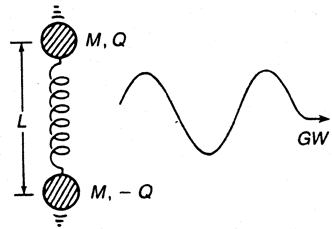For example, consider a gravitational wave propagating in the z direction in Cartesian coordinates. The wave only deforms space-time in the direction perpendicular to its propagation direction. Deformation of space-time means shrinking and stretching of the physical length between two points. Gravitational waves affect space-time in such a way that certain deformation patterns occur periodically.

If at time t, space is stretched in the x-direction at the maximum amplitude, then at time t+T/2 (where T is the period), one-half period later, space will have shrunk by the maximum amplitude and then will again stretch to its maximum at time t+T, one full period later. The theory also states that in the y-direction, space is deformed in the opposite phase of the x-direction. Thus, if space is stretched out in the x-direction, space is shrunk in the y-direction.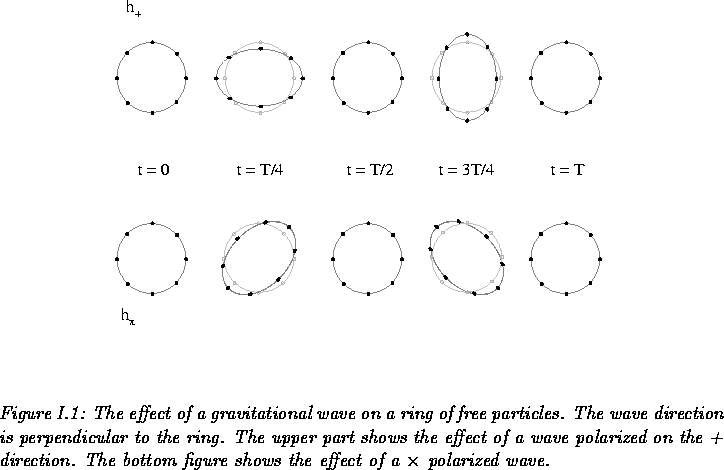## Stretching and Shrinking

The relative length change of two points resulting from gravitational wave is expressed as

Max stretching & shrinking = hL,
where L is a distance between two points and h is the dimensionless strain amplitude which is proportional to the second derivative of the mass distribution of the source and inversely proportional to the distance from the source. Therefore, the ratio of the stretched length to the original length is the same for any two points if the measurements were exercised at the same angle and distance from the source. However, the longer the original length, the larger the actual stretching/shrinking effect. This is why interferometers are as large as 4km and larger ones are being developed. The fact is that motion of the existing matter in the universe limits h to the range of less than 10^(-21). A 10km interferometer will be able to detect length changes of 10^(-17)m, which is certainly technically challenging since various sources of noise make it difficult to distinguish the gravitational wave. Gravitational waves have not yet been directly detected by this method.

## Sources of detectable gravitational wave

• ### Binary system

The magnitude of the gravitational waves depends on the distance from the source and the second derivative of the mass distribution (mass times acceleration in case of one particle). Thus, one must consider very massive objects moving violently as the candidates for the sources of detectable gravitational waves. The most promising candidates are compact binaries consisting of either two neutron stars, two black holes or one neutron star and one black hole. They are small and heavy, which allows them to orbit at a closer distance and at a high orbital frequency, which means that the second derivative of the mass distribution of the system is large. Therefore, the system emits strong gravitational waves. Compact binaries have another interesting aspect associated with gravitational radiation (explained in a later section).

• ### Rotating neutron stars

If non axis-symmetric along the rotation axis, a rotating neutron star will emit gravitational waves. If symmetric, the second derivative of the mass distribution holds constant at zero in the system, which leads to no gravitational wave emission.

• ### Supernovae

Supernovae are a good gravitational source. They are compact and have large accelerations. Similar to rotating neutron stars, if a supernova's explosion has axial symmetry, gravitational waves will not be emitted for the constant mass distribution. Initial density and temperature fluctuations and other factors may direct asymmetric collapse. If a supernova, which is exceptionally bright, is observed in gravitational wave, we will be able to test a prediction of general relativity which states that the speed of gravitational wave is the same speed as light.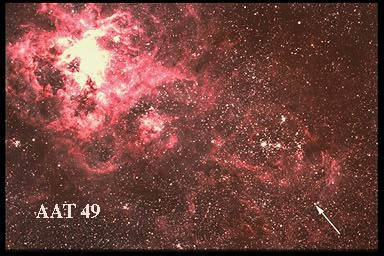• ### Stochastic background

This comes from the density fluctuation of the early stage of the universe. Measuring the background would tell us about the nature of the Plank-size universe and provide clues for testing the various cosmological models. Although stochastic background is interesting, it is so weak that modern technology is far from achieving this task.

## Relation between the motion of the source and the period and the amplitude of the consequent gravitational wave

The magnitude of gravitational waves is proportional to the second derivative of the mass distribution of the emitting system and inversely proportional to the separation of the source and observing point. The next question that arises is how the period of a gravitational wave is related to that of the motion of the source. If the binaries are in a circular orbit, the resulting gravitational waves have a frequency that is twice that of the binary system--that is, the period of the gravitational wave is one half of the orbital period.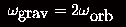The magnitude changes with the orientation. However an angle-averaged estimate of the signal strength is expressed in the following formula.

missing formula

To see why this is proportional to the second derivative of the mass distribution of the system, recall that the derivative is proportional to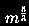and inversely proportional to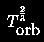.

This leads to the above equation. Therefore, if the frequency of the orbital period increases, the resulting gravitational wave will also increase its frequency and amplitude. And this is achieved by self-energy loss of gravitational radiation.

## Gravitational radiation and self-energy loss of binary system

According to general relativity, gravitational radiation takes energy from its source. The energy loss results in the smaller separation of the binaries and therefore shorter orbital frequency. This comes from the fact that the rate of change in orbital period of a binary system is negatively proportional to the rate of change in gravitational potential energy of the binary system: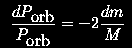,
where m is the gravitational mass of the primary star and M is the total gravitational mass of the binary system. The gravitational mass m is the sum of the rest mass and the total self energy of the gravitating body.

As the system radiates gravitational energy, it decreases its orbital period. Therefore, the resulting gravitational wave has a waveform of increasing frequency and amplitude. Furthermore, the radiation energy is proportional to the square of the amplitude of the associated gravitational wave. This means that the radiation power increases faster and faster as the system loses its energy due to gravitational radiation and thus resulting in the larger rate of change in orbital period. In fact, the final stage of the binary system consisting of two neutron stars has such a large radiation energy that the neutron stars will lose all their potential energy and finally collide. The waveform resulting from the collision will be very rough. Looking at the waveform, we can also obtain information about the mechanism of astronomical events.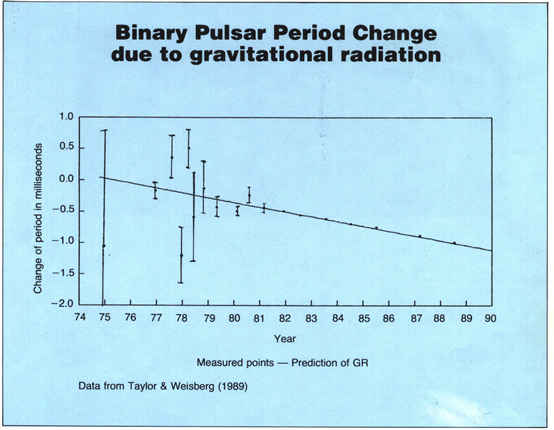Collision of two neutron stars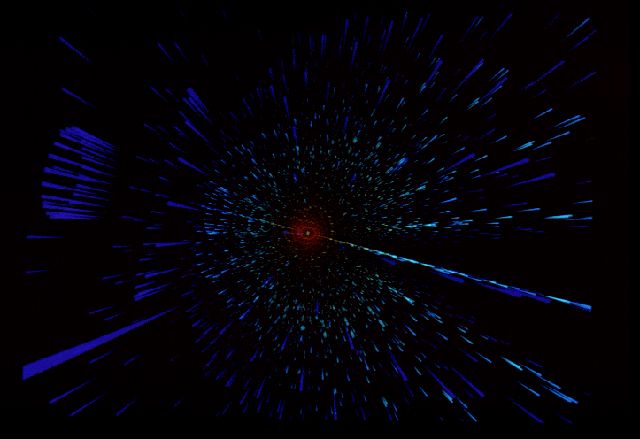Binary Neutron Star Merger, calculated by Maximilan Ruffert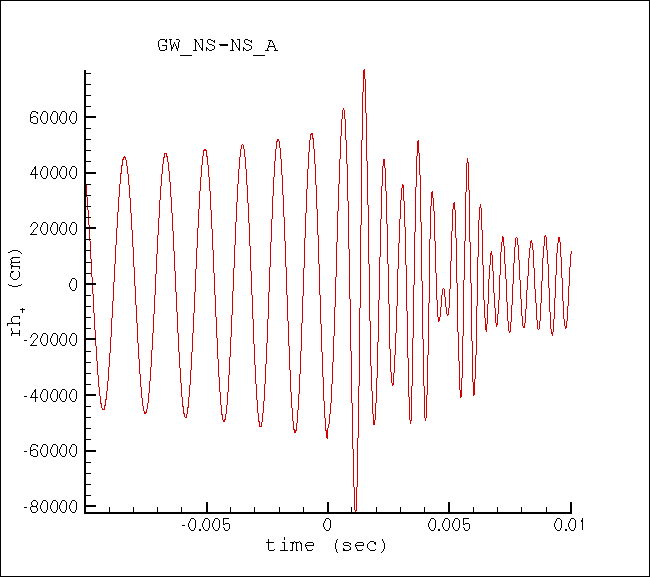Black Hole - Neutron Star Binary Mergers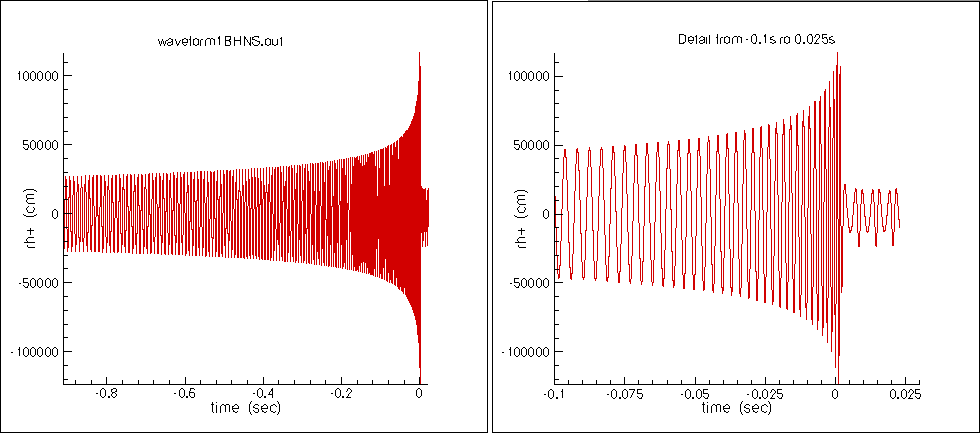## Why is it important to measure the gravitational wave?

Gravitational waves are most unique in that they propagate without interacting with matter. This allows us to obtain new information about the universe that electromagnetic waves fail to provide. The amplitude and frequency of gravitational waves describe the frequency and mass of the emitting source. The shape of the final phase of a binary system might give some new insight in astronomy. Stochastic background would reveal the mass distribution of the early plank-scale universe and the evolution of the early universe.